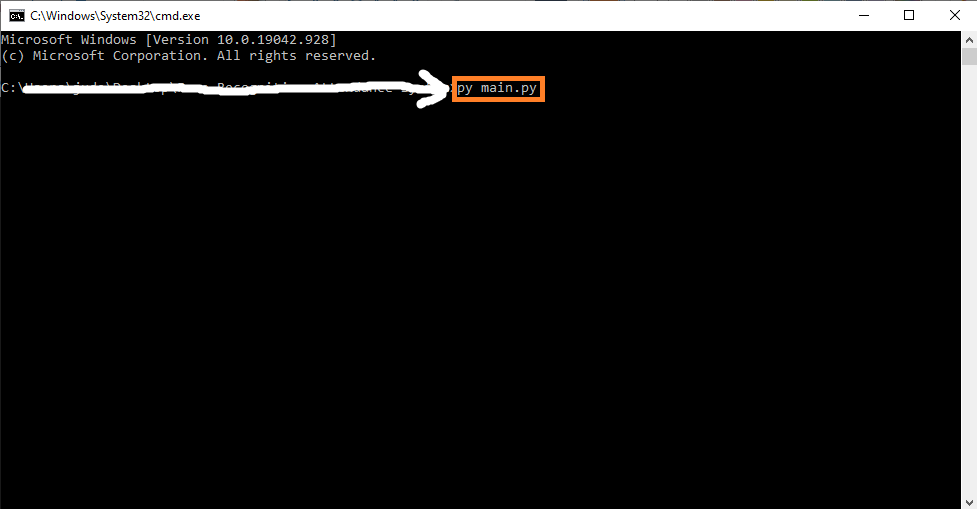# Weather Forecast Project In Python With Source Code

## Weather Forecast Project In Python With Source Code

The Weather Forecast Project In Python was developed using Python Programming, this Simple Project With Source Code created using console based, and this project is good for the beginners or the students who wants to learn programming specially python programming language.

A Weather Prediction Project In Python is a simple console based application using machine learning which helps to determine if the current situation of weather is good to play or not. It is done under the supervised learning in which data are given first to train the system and then the result for new data will be produce.

By the way if you are new to python programming and you don’t know what would be the the Python IDE to use, I have here a list of Best Python IDE for Windows, Linux, Mac OS that will suit for you. I also have here How to Download and Install Latest Version of Python on Windows.

To start executing Weather Forecast Project In Python With Source Code, make sure that you have installed Python 3.9 and PyCharm in your computer.

## Weather Forecast Project In Python With Source Code : Steps on how to run the project

Time needed: 5 minutes

These are the steps on how to run Weather Forecast Project In Python With Source Code

First, download the given source code below and unzip the source code.• Step 2: Import the project to your PyCharm IDE.• Step 3: Run the project.

last, run the project with the command “py main.py”## Installed Libraries

```from functools import reduce
import pandas as pd
import pprint```

## Complete Source Code

```from functools import reduce
import pandas as pd
import pprint

class Classifier():
data = None
class_attr = None
priori = {}
cp = {}
hypothesis = None

def __init__(self,filename=None, class_attr=None ):
self.class_attr = class_attr

'''
probability(class) =    How many  times it appears in cloumn
__________________________________________
count of all class attribute
'''
def calculate_priori(self):
class_values = list(set(self.data[self.class_attr]))
class_data =  list(self.data[self.class_attr])
for i in class_values:
self.priori[i]  = class_data.count(i)/float(len(class_data))
print ("Priori Values: ", self.priori)

'''
Here we calculate the individual probabilites
P(outcome|evidence) =   P(Likelihood of Evidence) x Prior prob of outcome
___________________________________________
P(Evidence)
'''
def get_cp(self, attr, attr_type, class_value):
data_attr = list(self.data[attr])
class_data = list(self.data[self.class_attr])
total =1
for i in range(0, len(data_attr)):
if class_data[i] == class_value and data_attr[i] == attr_type:
total+=1

'''
Here we calculate Likelihood of Evidence and multiple all individual probabilities with priori
(Outcome|Multiple Evidence) = P(Evidence1|Outcome) x P(Evidence2|outcome) x ... x P(EvidenceN|outcome) x P(Outcome)
scaled by P(Multiple Evidence)
'''
def calculate_conditional_probabilities(self, hypothesis):
for i in self.priori:
self.cp[i] = {}
for j in hypothesis:
self.cp[i].update({ hypothesis[j]: self.get_cp(j, hypothesis[j], i)})
print ("\nCalculated Conditional Probabilities: \n")
pprint.pprint(self.cp)

def classify(self):
print ("Result: ")
for i in self.cp:
print (i, " ==> ", reduce(lambda x, y: x*y, self.cp[i].values())*self.priori[i])

'''    Exit from the system it the input is "x" or "exit"   '''
def exitSystem():
print("System Terminated!")
print("Thank you for using this system!")
exit()

if __name__ == "__main__":
c = Classifier(filename="dataset.csv", class_attr="Play")
print("Enter the correct values shown in the option! *Case Sensitive")
print("Enter 'x' or 'exit' to exit from the system")
outlook = input("Whats the weather outside? (Sunny, Rainy, Overcast):")
if outlook.lower() == 'x' or outlook.lower() == 'exit':
exitSystem()
temp = input("Whats the temperature today? (Hot, Mild, Cool):")
if temp.lower() == 'x' or temp.lower()== 'exit':
exitSystem()
humidity = input("Whats the humidity? (High, Normal):")
if humidity.lower() == 'x' or humidity.lower()== 'exit':
exitSystem()
windy = input("Is it windy tody? (t or f):")
if windy.lower() == 'x' or windy.lower()== 'exit':
exitSystem()

c.hypothesis = {"Outlook":outlook, "Temp":temp, "Humidity":humidity , "Windy":windy}
c.calculate_priori()
c.calculate_conditional_probabilities(c.hypothesis)
c.classify()
```

## Summary

A simple machine learning project done in Python. This is a simple console based application using machine learning which helps to determine if the current situation of weather is good to play or not. It is done under the supervised learning in which data are given first to train the system and then the result for new data will be produce.

## Inquiries

1.